## Math in Focus Grade 7 Course 2 A Chapter 2 Lesson 2.1 Answer Key Adding Integers

### Math in Focus Grade 7 Chapter 2 Lesson 2.1 Guided Practice Answer Key

Copy and complete.

Question 1.
Evaluate -3 + (-2).
Method 1
Use a number line to model the sum of two negative integers.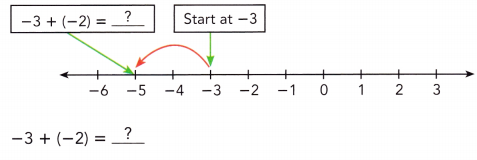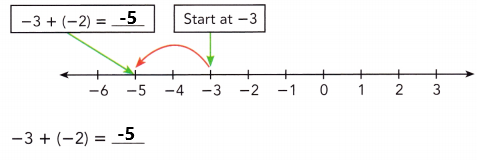Start at -3. Then add -2. Because you are adding a negative integer, -2, you make a jump of 2 to the left.Method 2
Use absolute values to find the sum of two negative integers.
|-3| =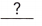Write the absolute value of each integer.
|-2| =|-3| + |-2| =+Add the absolute values.
=Simplify.
-3 + (-2) =Use the common sign, asign, for the sum.
|-3| = 3
|-2| = 2
|-3| + |-2| = 3 + 2
= 5
-3 + (-2) = -5 Used the common sign, a negative sign, for the sum.

Question 2.
Evaluate -15 + (-7).
Method 1
Use a number line to model the sum of two negative integers.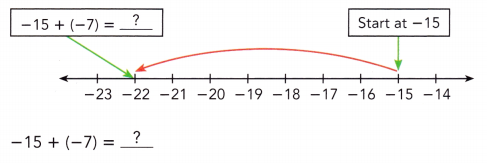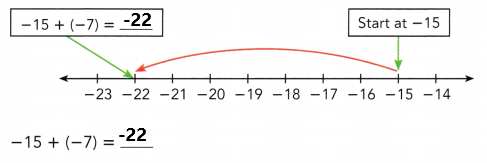Start at -15 then add -7. Because we are adding a negative integer, -7, we make a jump of 7 to the left.
Method 2
Use absolute values to find the sum of two negative integers.
|-15| =|-7| =|-15| + |-7| =+=-15 + (-7) =|-15| = 15
|-7| = 7
|-15| + |-7| = 15 + 7
= 22
-15 + (-7) = -22

Evaluate each sum.

Question 3.
9 + (-9)
9 – 9 = 0
Explanation:
Perform addition operation on above two numbers 9 and -9. Add 9 with -9 the sum is o.

Question 4.
-21 + 21
-21 + 21 = 0
Explanation:
Perform addition operation on above two numbers -21 and 21. Add -21 with 21 the sum is o.

Hands-On Activity

Materials:

• counters

Find the sum of integers with different signs.

Work in pairs.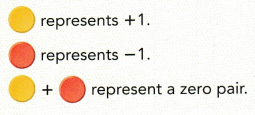A zero pair has a value of zero.
(+1) + (-1) = (-1) + (+1)
= 0
Removing a zero pair does not change the value of an expression. For example:
(+1) + (-1) + 2 = 2 because
(+1) + (-1) + 2 = 0 + 2
= 2

Step 1.
Use counters to model and find the sum of two integers with different signs.
a) Evaluate 3 + (-2).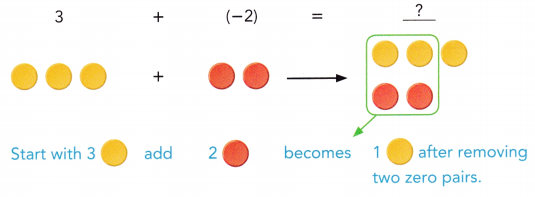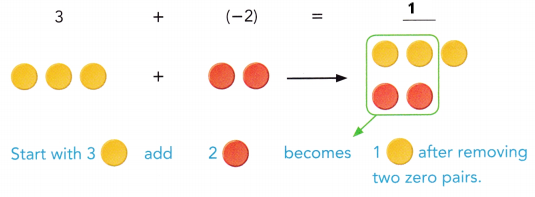A zero pair has a value of zero.
(+2) + (-2) = (-2) + (+2)
= 0
Removing a zero pair does not change the value of an expression.
(+2) + (-2) + 1 = 1 because
(+2) + (-2) + 1 = 0 + 1
= 1

b) Evaluate (-3) + 2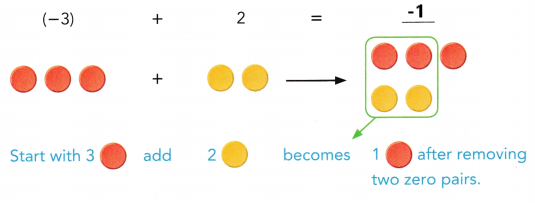A zero pair has a value of zero.
(+2) + (-2) = (-2) + (+2)
= 0
Removing a zero pair does not change the value of an expression.
(-2) + (+2) + (-1)  = -1 because
(-2) + (+2) + (-1) = 0 + (-1)
= -1

Step 2.
Use counters to model and find each sum.
a) 7 + (-2) and (-7) + 2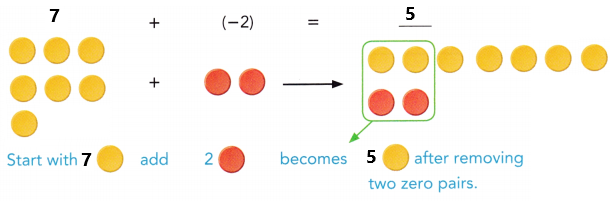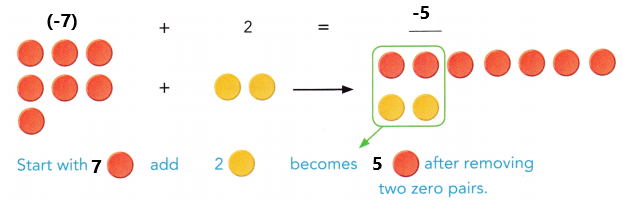b) (-8) + 5 and 8 + (-5)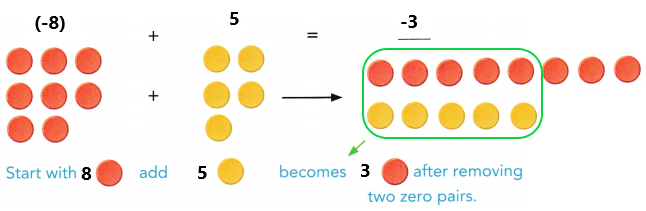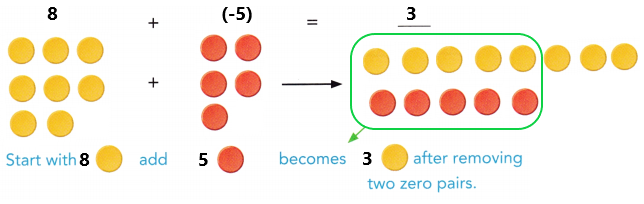Math Journal
Explain how to add two integers with different signs. How are the absolute values of the addends related to the sum?

Evaluate each sum.

Question 5.
-10 + 3
-10 + 3 = -7
Explanation:
Perform addition operation on above two numbers -10 and 3 the sum is -7.

Question 6.
-9 + 2
-9 + 2 = -7
Explanation:
Perform addition operation on above two numbers -9 and 2 the sum is -7.

Question 7.
11 + (-23)
11 + (-23) = -12
Explanation:
Perform addition operation on above two numbers 11 and -23 the sum is -12.

Evaluate each sum.

Question 8.
10 + (-3) + 6
10 + (-3) + 6
= 16 + (-3)
= 13
Explanation:
Perform addition operation on above numbers. First add 10 with 6 the sum is 16. Next add the sum 16 with -3 the sum is 13.

Question 9.
-7 + (-23) + 15
-7 + (-23) + 15
= -30 + 15
= -15
Explanation:
Perform addition operation on above numbers. First add -7 with -23 the sum is -30. Next add the sum -30 with 15 the sum is -15.

Solve.

Question 10.
A submarine ¡s at 400 feet below sea level. If it ascends 150 feet and then descends 320 feet, how far is ¡t above or below sea level?

You can think of the submarine ascending as an adding a positive integer, and descending as adding a negative integer. So, the verbal description can be translated as -400 + 150 + (-320).-400 + 150 + (-320) = -570
A submarine is 570 feet below sea level.
Explanation:
The submarine ascending as an adding a positive integer, and descending as adding a negative integer. A submarine ¡s at 400 feet below sea level. It ascends 150 feet and then descends 320 feet. Add -400 with 150 and -320 the sum is -570 feet.

### Math in Focus Course 2A Practice 2.1 Answer Key

Evaluate each sum using a number line.

Question 1.
-3 + (-9)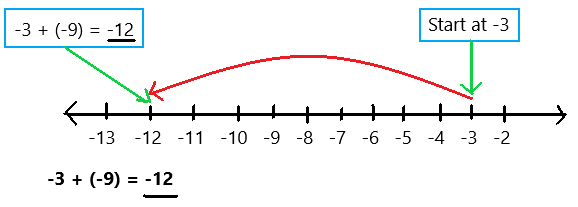Explanation:
To evaluate the sum of two negative integers use a number line as we can observe in the above image. Start at -3. Then add -9. Because i am adding a negative integer, -9, I make a jump of 9 to the left. The sum of two negative integers is -12.

Question 2.
-8 + (-4)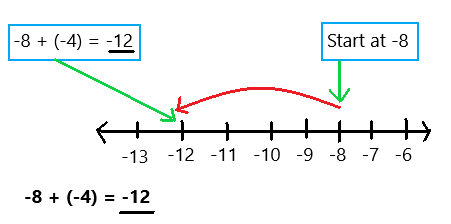Explanation:
To evaluate the sum of two negative integers use a number line as we can observe in the above image. Start at -8. Then add -4. Because i am adding a negative integer, -4, I make a jump of 4 to the left. The sum of two negative integers is -12.

Question 3.
7 + (-7)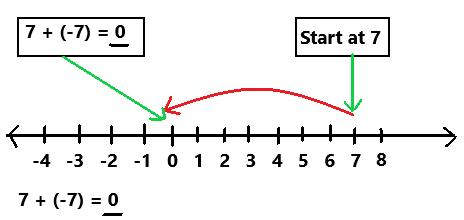Explanation:
To evaluate the sum of two integers use a number line as we can observe in the above image. Start at 7. Then add -7. Because i am adding a negative integer, -7, I make a jump of 7 to the left. The sum of two integers is 0.

Question 4.
-9 + 9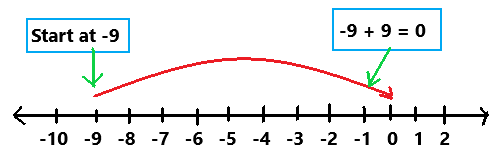To evaluate the sum of two integers use a number line as we can observe in the above image. Start at -9. Then add 9. Because i am adding a positive integer, 9, I make a jump of 9 to the right. The sum of two integers is 0.

Question 5.
-10 + 6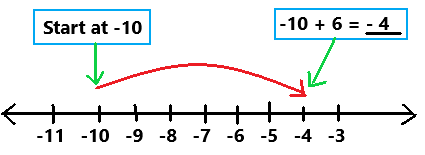Explanation:
To evaluate the sum of two integers use a number line as we can observe in the above image. Start at -10. Then add 6. Because i am adding a positive integer, 6, I make a jump of 6 to the right. The sum of two integers is -4.

Question 6.
-17 + 9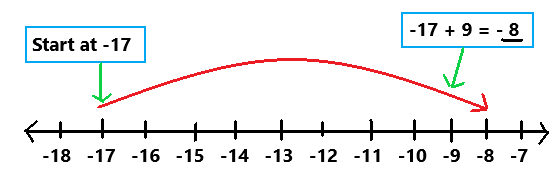Explanation:
To evaluate the sum of two integers use a number line as we can observe in the above image. Start at -17. Then add 9. Because i am adding a positive integer, 9, I make a jump of 9 to the right. The sum of two integers is -8.

Evaluate each sum using the absolute values.

Question 7.
-23 + (-9)
Use absolute values to find the sum of above two negative integers.
|-23| = 23
The absolute value of -23 is 23.
|-9| = 9
The absolute value of -9 is 9.
|-23| + |-9| = 23 + 9
= 32
-23 + (-9) = -32
Use the common sign, a negative sign, for the sum.

Question 8.
-11 + (-34)
Use absolute values to find the sum of above two negative integers.
|-11| = 11
The absolute value of -11 is 11.
|-34| = 34
The absolute value of -34 is 34.
|-11| + |-34| = 11 + 34
= 45
-11 + (-34) = -45
Use the common sign, a negative sign, for the sum.

Question 9.
-15 + (-7)
Use absolute values to find the sum of above two negative integers.
|-15| = 15
The absolute value of -15 is 15.
|-7| = 7
The absolute value of -7 is 7.
|-15| + |-7| = 15 + 7
= 22
-15 + (-7) = -22
Use the common sign, a negative sign, for the sum.

Question 10.
12 + (-18)
Use absolute values to find the sum of above two integers.
|12| = 12
The absolute value of 12 is 12.
|-18| = 18
The absolute value of -18 is 18.
|12| + |-18| = 12 + 18
= 30
12 + (-18) = -6
Use the common sign, a negative sign, for the sum.

Question 11.
-40 + 26
Use absolute values to find the sum of above two integers.
|-40| = 40
The absolute value of -40 is 40.
|26| = 26
The absolute value of 26 is 26.
|-40| + |26| = 40 + 26
= 66
-40 + 26 = -14
Use the common sign, a negative sign, for the sum.

Question 12.
-75 + 19
Use absolute values to find the sum of above two integers.
|-75| = 75
The absolute value of -75 is 75.
|19| = 19
The absolute value of 19 is 19.
|-75| + |19| = 75 + 19
=94
-75 + 19 = -56
Use the common sign, a negative sign, for the sum.

Evaluate each sum.

Question 13.
-8 + 4 + 5
-8 + 4 + 5
= -8 + 9
= 1
Explanation:
Perform addition operation on above numbers. First add 4 with 5 the sum is 9. Next add -8 with 9 the sum is 1.

Question 14.
5 + (-10) + (-6)
5 + (-10) + (-6)
= -5 + (-6)
= -11
Explanation:
Perform addition operation on above numbers. First add 5 with -10 the sum is -5. Next add -5 with -6 the sum is -11.

Question 15.
-6 + (-8) + (-12)
-6 + (-8) + (-12)
= -14 + (-12)
= -26
Explanation:
Perform addition operation on above numbers. First add -6 with -8 the sum is -14. Next add -14 with -12 the sum is -26.

Question 16.
-13 + (-17) + 7
-13 + (-17) + 7
= -30 + 7
= -23
Explanation:
Perform addition operation on above numbers. First add -13 with -17 the sum is -30. Next add -30 with 7 the sum is -23.

Question 17.
-20 + 16 +(-7)
-20 + 16 +(-7)
= -4 + (-7)
= -11
Explanation:
Perform addition operation on above numbers. First add -20 with 16 the sum is -4. Next add -4 with -7 the sum is -11.

Question 18.
-11 + (-8) + 14
-11 + (-8) + 14
= -19 + 14
= -5
Explanation:
Perform addition operation on above numbers. First add -11 with -8 the sum is -19. Next add -19 with 14 the sum is -5.

Question 19.
The temperature is originally recorded as -4°F. What will the temperature be if the temperature rises 20°F?
-4°F + 20°F = 16°F
Explanation:
The temperature is originally recorded as -4°F. The temperature rises to 20°F. Add -4°F with 20°F the sum is 16°F. The temperature is 16°F.

Question 20.
Mr. Lawson parked his car in a parking garage 33 feet below street level. He then got in an elevator and went up 88 feet to his office. How far above street level is his office?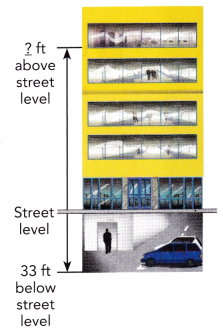88 – 33 = 55 feet
His office is 55 feet above street level.
Explanation:
Mr. Lawson parked his car in a parking garage 33 feet below street level. He then got in an elevator and went up 88 feet to his office. Subtract 33 feet below street level from 88 feet the difference is 55 feet. His office is 55 feet above street level.

Question 21.
A hiker starts hiking in Death Valley at an elevation of 143 feet below sea level. He climbs up 400 feet in elevation. What is his new elevation relative to sea level?
A hiker starts hiking in Death Valley at an elevation of 143 feet below sea level. Below sea level is represented with negative number which is -143 feet.
He climbs up 400 feet in elevation. The word ‘climbs up’ represents positive number which is 400 feet.
– 143 feet + 400 feet = 257 feet
His new elevation is 257 feet above seal level.

Question 22.
Elizabeth was playing a board game with her friends. On her first turn, she moved 6 spaces forward. On her second turn, she moved another 5 spaces forward. On her third turn, she moved 4 spaces backward. How many spaces forward or backward from her starting point was she after her third turn?
6 + 5 – 4
= 11 – 4
= 7
After her third turn Elizabeth moved 7 spaces forward from her starting point.
Explanation:
Elizabeth was playing a board game with her friends. On her first turn, she moved 6 spaces forward. Forward spaces are represented with positive number. On her second turn, she moved another 5 spaces forward. Forward spaces are represented with positive number. Add 6 with 5 the sum is 11. On her third turn, she moved 4 spaces backward. Backward spaces are represented with negative number. Subtract 4 from 11 the difference is 7. After her third turn Elizabeth moved 7 spaces forward from her starting point.

Question 23.
In the U.S. Open Golf Tournament, each qualifying golfer plays four rounds. The score for a round is recorded as positive (over par) or negative (under par). If a golfer scores -4, 6, 3, and -2 in the four rounds, what is the golfer’s total score for the tournament?
The golfer scores -4, 6, 3, and -2 in four rounds.
-4 + 6 + 3 -2
= 9 – 6
= 3
The golfer’s total score for the tournament is 3.

Question 24.
Math Journal
In a game, all scores with even numbers are recorded as positive numbers. Odd numbers are recorded as negative numbers. Explain how to find David’s total score in this game if his individual scores during the game are 9, 12, 7, 18, and 19.